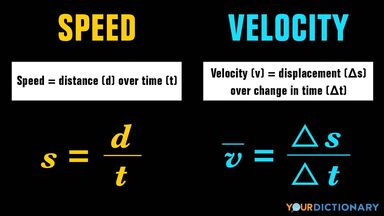# Main Difference Between Speed and Velocity

It may seem like the terms speed and velocity mean the same thing. But if you’re studying physics, it’s important to know the difference between these two concepts. Keep reading to learn the main difference between speed and velocity and what information their measurements can tell us.difference between speed and velocity formula

## How to Tell Speed and Velocity Apart

Speed and velocity both measure an object’s rate of motion. However, speed is a scalar quantity, which means that it can be described with a numerical value. Velocity is a vector quantity, which depends on direction as well as magnitude. Read these definition for more information:

• speed - the rate of distance traveled by a moving object over time
• velocity - the rate of displacement of a moving object over time

Basically, an object’s speed tells you how fast it’s going. Its velocity tells you how fast it’s going in a certain direction. You use speed measurements in your daily life, but physicists depend on velocity measurements more frequently in their work.

## How Speed Works

There are two ways to measure an object’s speed: its average speed and its instantaneous speed. The average speed tells you the average measurement of how fast it was going over a period of time. Its instantaneous speed tells you how fast it’s going in a single instance.

There are three units to measure speed:

• m/s - meters per second (used by The International System of Units and Measurements)
• kph - kilometers per hour (used by countries that use the metric system)
• mph - miles per hour (used in the United States)

There are several tools that measure an object’s speed. Tachometers measure an engine’s speed in rpms (revolutions per minute) and speedometers measure the speed in an automobile. RADAR can also measure the speed of a moving object from a distance.

## How Velocity Works

Velocity depends on the direction an object travels. It incorporates the speed of that object as well as the difference between its starting place and its ending place. You use the same units for speed (m/s, kph, and mph) when measuring velocity, but factoring in its direction.

You need to calculate the velocity of objects when determining where they might go. Like speed measurements, velocity can be measured by an object’s average velocity and its instantaneous velocity.

## Equations for Speed and Velocity

It’s easiest to see the difference between speed and velocity when you compare their equations. In order to measure an object’s speed (s), you measure its distance (d) over time (t):

s = d/t

Once you know the object’s speed, you can calculate its average velocity by calculating the change in displacement (d) over the change in time (t):

v = Δs/Δt

This information may not seem as straightforward for an everyday traveler, but when studying physics, it’s very important to know the difference between an object’s speed and velocity. When they are the same value, the object has traveled in a straight line. Different values between speed and velocity indicate that the object’s path was not straight.